# Trapping Rain Water in a Matrix

## Introduction

What do you mean by a matrix? Matrix is a two-dimensional structure identified by two indices, one for rows and another for columns. Every matrix is a two-dimensional array but not vice versa.

Trapping rainwater is a famous and routine question asked in interviews of many companies like Google, Amazon, Samsung, Adobe, etc. This particular problem we will be heading to is of the topic matrix. Matrix will contain the height of each cell that can hold the rainwater, and we have to return the total volume of water it can trap after raining.

Let’s move to our problem statement for better understanding so that you can think of the appropriate approach to solve the problem.

## Problem Statement

So the problem statement is pretty simple, we are given an mxn matrix of positive integers where each element of the matrix represents the height of the bars; we aim to find the total volume of water trapped in the matrix after raining.

Let’s understand the idea of how to solve this problem through an example.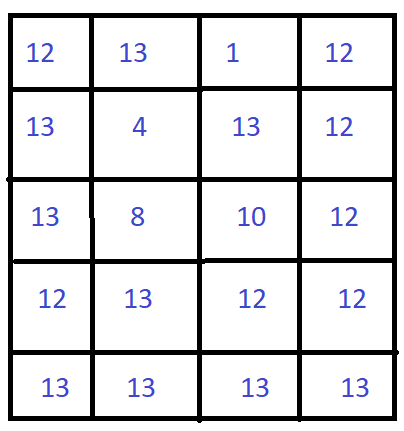This is like a container, and the water-filled in the container will be till the minimum boundary.

So in this container, first, I will be processing the outside boundaries because everything will be stored inside these boundaries, and slowly, I will push in the boundary.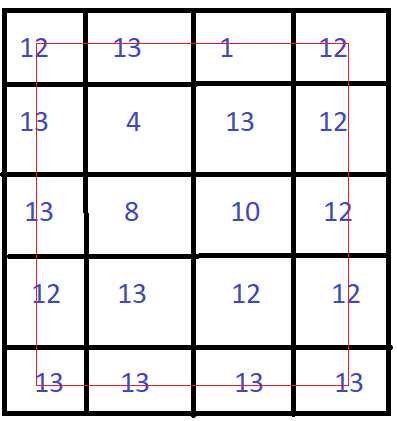After visiting the boundary, we can see that the minimum value we are getting from here is ‘1’.

After visiting ‘1’, we will reduce the boundary and include ‘13’ into the boundary.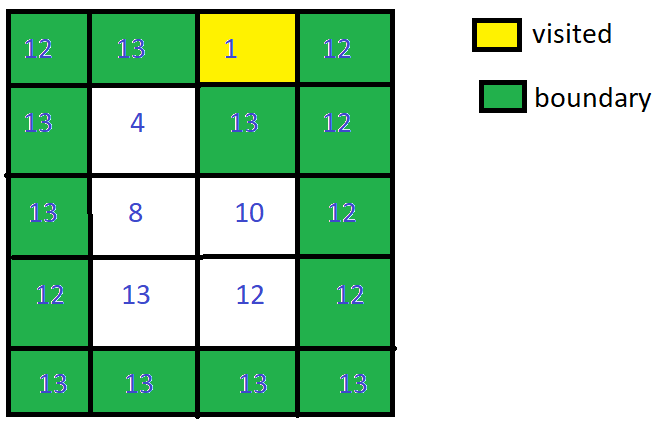The minimum boundary value is ‘12’ and updates the minimum value from ‘1’ to ‘12’.

We will push the boundary by checking the adjacent of each boundary having a value as ‘12’. If the adjacent ‘12’ is already inside the boundary, it will do nothing; otherwise, add the boundary if the boundary value is greater than ‘12’.

If the adjacent boundary value is less than ‘12’, then water will be filled inside that boundary. The amount of water-filled will be the minimum value of boundary - value of adjacent boundary(12-10=2).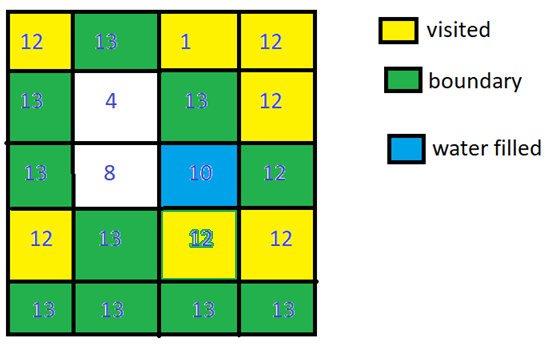The adjacent boundary of ‘10’ left outside is also less than ‘12’, i.e., ‘8’. So the amount of water filled will be 12 - 8 = 4.

The adjacent boundary of ‘8’ left outside the boundary is also less than ‘12’, i.e., ‘4’. So the amount of water filled will be 12 - 4 = 8.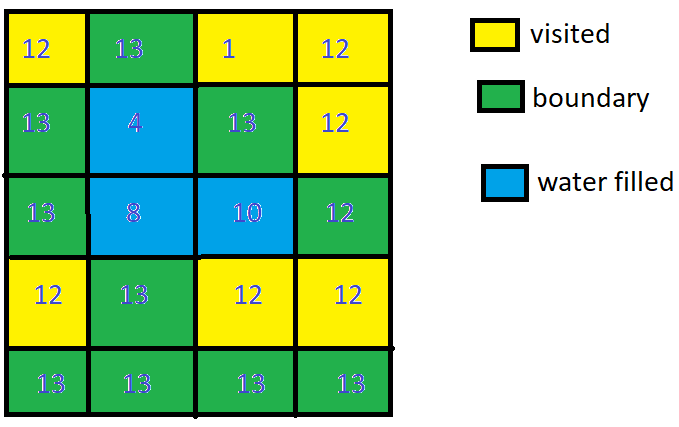The total amount of rainwater trapped will be 2+4+8=14

Now the minimum boundary value will get updated to ‘13’ and check for the adjacent boundaries that are unvisited or visited.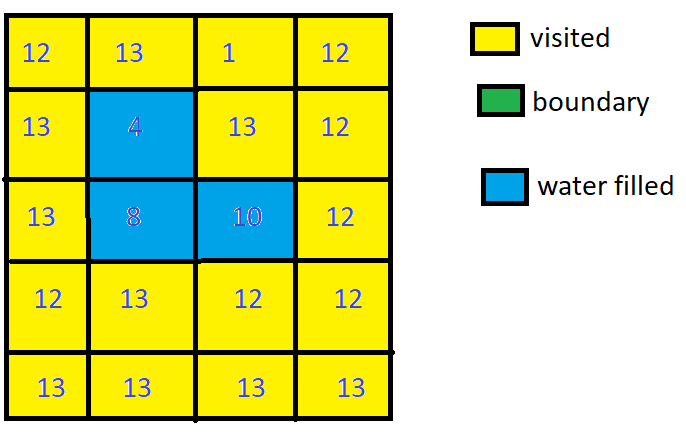We can see that all the adjacent boundaries of ‘13’ are visited. So will get the total volume of rainwater trapped in between the bars after raining.

Note: Please try to solve Trapping rainwater in a matrix on CodeStudio before heading into the solution.

## Approach: Priority Queue

Priority queue gonna help us to store the minimum value of the boundary. Every element in the priority queue is assigned with some priority, which will help fetch the minimum value more efficiently.

### Implementation

``````import java.util.ArrayList;
import java.util.PriorityQueue;
import java.util.Queue;
import java.util.List;

public class Trappingrainwater1 {

// To define the coordinate of the cells
class Pair{
int x;
int y;
int height;
public Pair(int x, int y, int height){
this.x =x ;
this.y =y;
this.height = height;
}
}
public int trapRainWater(int[][] mat) {
// Base conditions
if(mat == null || mat.length == 0 || mat.length == 0)
return 0;

int m = mat.length;
int n = mat.length;

// To keep an update regarding the visited boundaries
boolean[][] visited = new boolean[m][n];
// To store the minimum value from the boundary
PriorityQueue<Pair> pq = new PriorityQueue<>((a,b)->(a.height - b.height));

// To process the boundaries
for(int i = 0; i<m;i++){
for(int j = 0; j<n; j++){
if(i==0 || j==0 || i == m-1 || j == n-1){
visited[i][j] = true;
pq.offer(new Pair(i,j,mat[i][j]));
}
}
}
// For the BFS traversal, we can go UP, DOWN, LEFT, and RIGHT.
// To explore the adjacent cells we are creating the 2D direction matrix.
int[][] dirs = new int[][]{{0,1},{1,0},{0,-1},{-1,0}};
// To store the total volume of rain water
int res = 0;
while(!pq.isEmpty()){
Pair cur = pq.poll();
for(int i = 0 ; i<dirs.length; i++){
int newX = cur.x + dirs[i];
int newY = cur.y + dirs[i];
if(newX < 0 || newY <0 || newX>=m || newY>=n || visited[newX][newY]){
continue;
}
visited[newX][newY] = true;
// When the neighour of the minimum boundary value is less than its value
res += Math.max(0,cur.height - mat[newX][newY]);
pq.offer(new Pair(newX,newY,Math.max(mat[newX][newY],cur.height)));
}

}
return res;
}
}``````

### Analysis of Complexity

Time Complexity: row:matrix height, col: matrix width --> step1: O(rowcol log(2m+2n-4)) = O(rowcol log(m+n)).

Space Complexity: boolean matrix and PriorityQueue--> O(m*n)

## FAQ’S

1). What is the motive behind the problem of Trapping rainwater in a matrix?

Trapping rainwater means finding the total volume of water stored between the bars after raining.

2). What do you mean by Priority Queue?

A priority queue is the extension of the queue data structure where each element is associated with some priority. Through priority, vital data gets through faster.

3). What is the time complexity to solve this problem?

Time Complexity: (row * col * log(col * row))

Auxiliary Space: O(col * row)

## Key Takeaways

In this article, we have covered the problem of Trapping rainwater in a matrix using a priority queue in the Java language. With the help of the pictorial representations and examples, it will be clear to you. You can practice more questions similar to this like Trapping Rain WaterContainer With Most Water, and many more.

If you are not familiar with priority queues, you can visit CodeStudio for more information.

For more practice, you can use CodeStudio for various DSA questions typically asked in interviews. It will help you in mastering efficient coding techniques.

Keep Coding!!!